# Revision Notes: Atomic Physics Notes | Study Mock Test Series for JEE Main & Advanced 2022 - JEE

## JEE: Revision Notes: Atomic Physics Notes | Study Mock Test Series for JEE Main & Advanced 2022 - JEE

The document Revision Notes: Atomic Physics Notes | Study Mock Test Series for JEE Main & Advanced 2022 - JEE is a part of the JEE Course Mock Test Series for JEE Main & Advanced 2022.
All you need of JEE at this link: JEE

Thomson Method

• Specific Charge (e/m) of an electron
(i) e/m of a particle is called the specific charge of the particle.
i.e. e/m = v/rB
Here, r is the radius of curvature, B is the strength of the magnetic field, v is the velocity, e is the charge on cathode ray particle and m is the mass.
(ii) v = E/B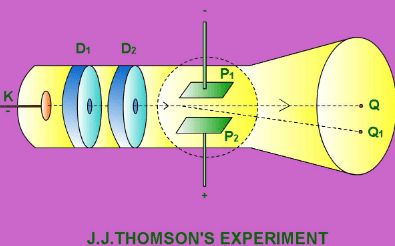• Electric field: E = V/d

What is Photoelectric effect?

The photo-electric effect is the phenomenon of emission of electrons from the surfaces of certain substances, mainly metals when the light of a shorter wavelength is incident upon them.

Effect of collector’s potential on the photoelectric current:

1. The presence of current for zero value potential indicates that the electrons are ejected from the surface of the emitter with some energy.
2. A gradual change in the number of electrons reaching the collector due to a change in its potential indicates that the electrons are ejected with a variety of velocities.
3. Current is reduced to zero for some negative potential of collector indicating that there is some upper limit to the energy of electrons emitted.
4. Current depends upon the intensity of incident light.
5. Stopping potential is independent of the intensity of light.

Try yourself:The phenomenon of photoelectric emission depends on

Effect of intensity of light:

The photoelectric current is directly proportional to the intensity of incident radiation.

Effect of frequency of light:

1. Stopping potential depends upon the frequency of light. The greater the frequency of light greater is the stopping potential.
2. Saturation current is independent of frequency.
3. The threshold frequency is the minimum frequency, that capable of producing the photoelectric effect.

Einstein’s equation of photoelectric effect

1. ½ mvmax2 = h– W0
2.  ½ mvmax= h– hf0 = h(f- f0) = h [c/λ – c/λ0]
3. eV0 = hf - W0
4. V0 = [(h/e)f] – [W0/e]
Here fis threshold frequency.

Threshold frequency (f0): f0 = work function/h = W/h

Laws of Photoelectricity

• The photoelectric effect is an instantaneous process.
• Photoelectric current is directly proportional to the intensity of incident light and is independent of its frequency.
• The stopping potential and hence the maximum velocity of the electrons depends upon the frequency of incident light and is independent of its frequency.
• The emission of electrons stops below a certain minimum frequency known as the threshold frequency.

Energy contained in bundle or packet:

E = hf = hc/λ
Here h is Planck’s constant and f is the frequency.

What is the Work function?

It is defined as the minimum energy required to pull an electron out from the surface of a metal. It is denoted by W0.

Important Terms & Equations:

• Maximum kinetic energy of emitted photo electrons: Kmax= ½ mvmax2 = eV0
• Threshold wavelength: λ= c/f0 = hc/hf0 = hc/W
• Slope of V0~ v graph: Slope= h/e
• Rest mass of photon = 0, Charge = 0
• Energy of photon: E = hf = hc/λ
• Momentum of photon: p = E/c = h/λ = hf/c
• Mass of photon: m = E/c= h/cλ = hf/c2
• For electron: λe = [12.27/√V]Å
• For proton: λp = [0.286/√V]Å
• For alpha particle: λα = [0.286/√V]Å
• For particle at temperature, T: λ = h/√3m KT   (E = 3/2 KT)
• The wavelength of electron accelerated by potential difference of V volts is:
λe= [12.27/√V]Å
• Number of photons
(i) Number of photons per sec per m2, n= Intensity/hf
(ii) Number of photons incident per second, np = Power/hf
(iii) Number of electrons emitted per second = (efficiency per surface) × (number of photons incident per second)
• Compton wave length
(i) λc = h/m0c
Here h is Planck’s constant, m0 is the rest mass of electron and c is the speed of light.
(ii) Change in wavelength: λ' – λ =λc (1-cosθ)
• De Broglie wavelength (λ): λ = h/mv = h/√(2mE) = h/√(2meV)
• In accordance to Bohr’s postulate of atomic structure, the angular momentum of an electron is an integral multiple of h/2π.
So, mvr = nh/2π
• Bragg’s diffraction law: 2dsinθ = nλ
Here λ is the wavelength of electron and d is distance between the planes.

Rutherford’s atomic model (α-particle scattering)

• Rutherford carried out an experiment on the bombardment of thin (10–4 mm) Au foil with high speed positively α - charged particles.
• He concluded that an atom consists of a Nucleus that is small in size but carries the entire mass i.e. contains all the neutrons and protons.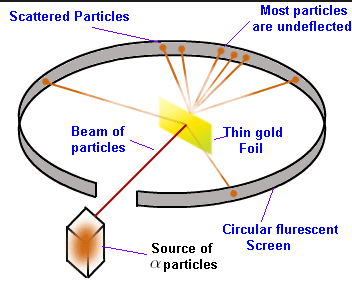•  N(θ) ∝ cosec4(θ/2)
• Impact parameter, b = [(Ze2) (cot θ/2)]/[(4πε0)E]
Here, E = ½ mv= KE of the α particle.
• Distance of closest approach, r0 = 2Ze2/(4πε0)E
Here E = ½ mv= KE of the α particle.

Properties of the nucleus:

• The small, heavy and positively charged portion of the atom is known as the nucleus. It is located at the centre of the atom and discovered in 1911 by Ernest Rutherford.
• Protons are positively charged particles.
• Neutrons and protons are present in the nucleus hence they are collectively known as nucleons.

Drawbacks of Rutherford's model:

• According to it “An electron moving around the nucleus continuously loses its energy and should set up spiral motion 2 ultimately failing into the nucleus”’. Hence this model is not able to explain how the moving electrons could remain in their orbit.
• It is not able to explain the discontinuous spectrum and the line spectra of the H - atom.

What is Planck's quantum theory?

According to this theory, the energy of each quanta is directly proportional to the frequency ( ϑ ) of the radiation, i.e.
E ∝ ϑ or E = hϑ; ϑ = c/λ.
Thus,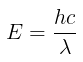Where, h is proportionality constant also known as Planck’s constant,h = 6.626 * 10-34 Joules sec or 6.626×10–27 erg sec

What is Bohr’s Atomic Model?

(i) The central part of the atom called nucleus, contains whole positive charge and almost whole the mass of atom. Electrons revolve round the nucleus in fixed circular orbits.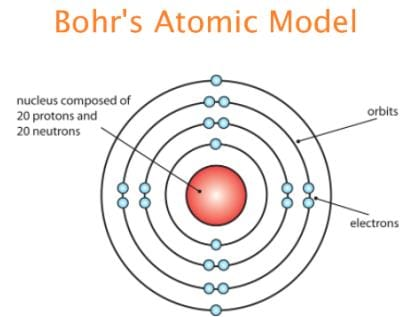(ii) Electrons are capable of revolving only in certain fixed orbits, called stationary orbits or permitted orbits. In such orbits they do not radiate any energy.
(iii) While revolving permitted orbit an electron possesses angular momentum (L = mvr) which is an integral multiple of h/2π.
L=mvr =n (h/2π)
Here n is an integer and h is Planck’s constant.
(iv) Electrons are capable of changing the orbits. On absorbing energy they move to a higher orbit while emission of energy takes place when electrons move to a lower orbit.
If f is the frequency of radiant energy, hf = W2-W1
Here W2 is the energy of electron in lower orbit and W1 is the energy of electron in higher orbit.
(v) All the laws of mechanics can be applied to electrons revolving in a stable orbit while they are not applicable to an electron in transition.

Bohr’s Theory of Atom

• Orbital velocity of electron: vn= 2πkZe2/nh
(i) For a particular orbit (n= constant), orbital velocity of electron varies directly as the atomic number of the substance, i.e. v∝ Z
(ii) For a particular element (Z= constant), orbital velocity of the electron varies inversely as the order of the orbit, i.e. v∝ 1/n
(iii) v = nh/2πmr

• Relation between vn and v1vn = v1/n

• Radius of electron: r = n2h2/4π2kmZe2
⇒ r ∝ n2
C.G.S system (k = 1), r = n2h2/4π2mZe2
S.I (k = 1/4πε0), r = (ε0/π) (n2h2/mZe2)

• Kinetic energy of the electron: It is the energy possessed by the electron by virtue of its motion in the orbit.
K.E. = ½ mv= ½ k (Ze2/r)

• Potential energy: It is the energy possessed by the electron by virtue of its position near the nucleus.
P.E = -k (Ze2/r )

• Total energy
W = K.E + P.E
W =- ½ k (Ze2/r) = -k22Z2me4/n2h2
For, C.G.S (k = 1), W = - [2π2Z2me4/n2h2]
For, S.I. ( k = 1/4πε0), W = - (1/8ε02) [Z2me4/n2h2]
Since, W∝1/n2, a higher orbit electron possesses lesser negative energy (greater energy) than that of a lower orbit electron.

• Frequency, wavelength and wavenumber of radiation
Frequency, f = k2[2π2Z2me4/h3] [1/n1– 1/n22]
Wavenumber of radiation,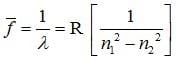Here R is the Rydberg’s constant and its value is: R = k2 [2π2Z2me4/ch3]

• Bohr’s theory of hydrogen atom (Z=1)
r = n2h4/4π2me(C.G.S)
r = (ε0/π) (n2h2/me2) (S.I)
(b) Energy of electron:
W = 2π2me4/n2h2    (C.G.S)
W = (1/8ε0)[me4/n2h2]
(c) Frequency, wavelength and wave number of radiation:
C.G.S: k = 1 and Z = 1
Frequency = f = 2π2me4/h[1/n12 – 1/n22]
Wave number = 1/λ = 2π2me4/ch3 [1/n12 – 1/n22]
S.I.: k = 1/4πε0 and Z = 1
Frequency = f = (1/8ε0) (me4/h3)[1/n12 – 1/n22]
Wave number = 1/λ = (1/8ε02) (me4/ch3)[1/n12 – 1/n22]

• Rydberg’s constant
R = k2 = 2π2z2 me4/ch3
For hydrogen atom, Z = 1, R = RH = k2 (2π2 me4/ch3).
For C.G.S system (k = 1), RH = 2π2 me4/ch3
For S.I system (k = 1/4πε0), RH = (1/8ε02) (me4/ch3)
Wave number, 1/λ = RH [1/n12 – 1/n22]

• Hydrogen Spectrum
(a) For Lyman series: 1/λ = R [1– 1/n2], n = 2,3,4…..∞
(b) For Balmer series: 1/λ = R [1/22 – 1/n2], n =3,4,5…..∞
(c) For Paschen series: 1/λ = R [1/32 – 1/n2], n =4,5,6…..∞
(d) For Brackett series: 1/λ = R [1/42 – 1/n2], n =5,6,7…..∞
(e) P-fund series: 1/λ = R [1/52 – 1/n2], n =6,7,8…..∞

• Series limits (λmin)
(a) Lyman: λmin = 912 Å
(b) Balmer: λmin = 3645 Å
(c) Paschen: λmin = 8201 Å

• Energy levels of hydrogen atom
W = -k22me4/n2h2
For, n=1, W= -13.6 eV
For the first excited state, n=2, W2 =W1/4 = (-13.6/4) eV = -3.4 eV
For the second excited state, n=3, W=W1/9 = (-13.6/9) eV = -1.51 eV
Similarly, for other excited states, W4 = -0.85 eV and W5 = -0.54 eV

• Number of emission lines from excited state: n = n(n-1)/2

• Ionization energy: - E= +(13.6Z2)eV
(a) For H-atom, I.E = 13.6 eV
(b) For He+ ion, I.E = 54.4 eV
(c) For Li++ ion, I.E = 122.4 eV

• Ionization potential
(a) For H-atom, I.P = 13.6 eV
(b) For He+ ion, I.P = 54.42 eV

Dual nature of electron

• According to the French physicist, Louis de Broglie, if light has an electron then it behaves as both a material particle and a wave.
• The wavelength associated with a particle of mass m, moving with velocity v is given by the relation,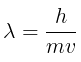,where h = Planck’s constant.
• According to Planck’s equation,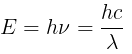on the basis of Einstein’s mass-energy relationship, E = mc2
on equating both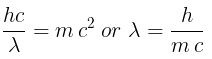which is the same as de-Broglie relation.(∵ mc = p)

Heisenberg’s uncertainty principle

This principle states “It is impossible to specify at any given moment both the position and momentum (velocity) of an electron”.

Mathematically it is represented as,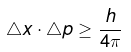Where Δx = uncertainty is position of the particle, Δp = uncertainty in the momentum of the particle.

Now since Δp  = mΔv

So equation becomes,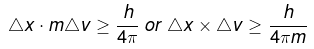In terms of uncertainty in energy, ΔE and uncertainty in time Δt, this principle is written as,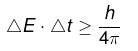Try yourself:The de-Broglie equation applies

Schrödinger wave equation

1. Schrodinger wave equation based on the dual nature of electron, is given by Erwin Schrödinger in 1926.
2. With the help of this wave equation, we can find the probability of an electron at any point around the nucleus. The probability of an electron at any point around the nucleus is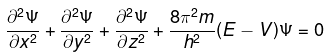Here, x, y and z are the 3 space co-ordinates, m is the mass of electron, h is  Planck’s constant, E is Total energy, V  is  potential energy of electron, Ψ is amplitude of wave also called as wave function and ∂ is an infinitesimal change.

What are Quantum numbers?

Orbital in an atom is specified by a set of three quantum numbers (n, l, m) and a set of four quantum numbers (n, l, m a nd s) is specified for each electron.

1. Principle quantum number (n)
The principal quantum number proposed by Bohr and denoted by ‘n’. It describes the energy of an electron in an orbit and the most probable distance between the electron and the nucleus. In other words, it denotes the size of orbital.
2. Azimuthal quantum number (l)
Azimuthal quantum number proposed by Sommerfield and denoted by ‘l’. It is also known as angular momentum quantum number or secondary quantum number. It describes the shape and number of the orbital(sub shells) to which the electron belongs. The value of l is always equals to (n -1) . Where 'n' is the number of principle shell.
Thus, the lowest possible value of l is 0, and its highest possible value,  is n - 1. Following values of l are given below.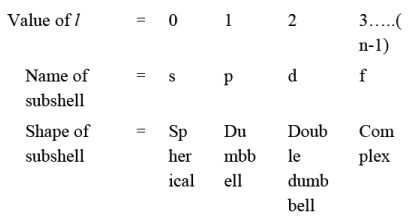It also represents the orbital angular momentum. That is equal to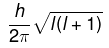3. Magnetic quantum number (m)
Magnetic quantum number denoted by ‘m’. It describes  the number of permitted orientation of the orbital(sub shell). The value of m varies from –l to +l through zero.  For a given value of ‘n’ the total value of ‘m = n2 ” ,  for a given value of ‘l’ the total value of ‘m = 2l +1" and the number of degenerate orbitals of s subshell = 0.
4. Spin quantum numbers (s)
Spin quantum number denoted by ‘m’.It signifies the spin or rotation or direction of electron on it’s axis during movement.
The value of it is either +1/2 or-1/2 . If the spin of electron is clockwise then value of S will be +1/2 and if its direction is anticlockwise then value of S will be -1/2 .
It represents the value of spin angular momentum. That is equal to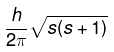Rules for filling of electrons in various orbitals

The atom is built up by filling electrons in various orbitals according to the following rules,

1. Aufbau’s principle
This principle states that the electrons are added one by one to the various orbitals in order of their increasing energy starting with the orbital of lowest energy. The increasing order of energy of various orbitals is
1s < 2s < 2p < 3s < 3p < 4s < 3d < 4p <5s < 4d <5p <6s < 4 <5d<6p<7s<5f <6d<7p.........
2. (n+l) Rule
In neutral isolated atom, the lower the value of (n + l) for an orbital, lower is its energy. However, if the two different types of orbitals have the same value of (n + l), the orbitals with lower value of n has lower energy.
3. Pauli’s exclusion principle
According to this principle “no two electrons in an atom will have same value of all the four quantum numbers”.
4. Hund’s Rule of maximum multiplicity
“Electron pairing in p, d and f orbitals cannot occur until each orbitals of a given subshell contains one electron each or is singly occupied”.
The document Revision Notes: Atomic Physics Notes | Study Mock Test Series for JEE Main & Advanced 2022 - JEE is a part of the JEE Course Mock Test Series for JEE Main & Advanced 2022.
All you need of JEE at this link: JEEUse Code STAYHOME200 and get INR 200 additional OFF

## Mock Test Series for JEE Main & Advanced 2022

2 videos|258 docs|160 tests

Track your progress, build streaks, highlight & save important lessons and more!

,

,

,

,

,

,

,

,

,

,

,

,

,

,

,

,

,

,

,

,

,

;